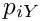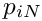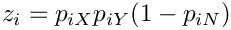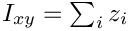Legofit infers population history from nucleotide site patterns.

Bootstrap model averaging

Bootstrap model averaging was proposed by Buckland et al (Biometrics, 53(2):603-618). It can be used with weights provided by any method of model selection, including bepe and clic. Model selection is applied to the real data and also to a set of bootstrap replicates. The weight,of the i'th model is the fraction of these data sets for which the i'th model wins. In other words, it is the fraction of data sets for which the i'th model has the smallest information criterion.

The model-averaged estimator of a parameter,, is the average across models, weighted by, of the model-specific estimates of. Models in whichdoes not appear are omitted from the weighted average.

To construct confidence intervals, we average across models within each bootstrap replicate to obtain a bootstrap distribution of model-averaged estimates.

Usage: booma <m1.msc> ... <mK.msc> -F <m1.flat> ... <mK.flat>

Here, the "mX" arguments refer to model "X". The "msc" suffix stands for "model selection criterion". There are currently two options: bepe and clic. Thus, the first command-line argument might look like either "m1.bepe" or "m1.clic".

In either case, the "msc" files consist (apart from sharp-delimited comments) of two columns. The first column gives the model selection criterion, and the second column names the data file to which that criterion refers. The first row should refer to the real data and the remaining rows to bootstrap replicates. Model selection criteria are defined so that low numbers indicate preferred models. I will refer to these numbers as "badness" values.

After the -F argument comes a list of files, each of which can be generated by flatfile.py. There must be a .flat file for each model, so the number of .flat files should equal the number of .bepe files. The first row of a .flat file is a header and consists of column labels. Each column refers to a different parameter, and the column labels are the names of these parameters. The various .flat files need not agree about the number of parameters or about the order of the parameters they share. But shared parameters must have the same name in each .flat file.

After the header, each row in a .flat file refers to a different data set. The first row after the header refers to the real data. Each succeeding row refers to a bootstrap replicate. The number of rows (excluding comments and the header) should agree with the numbers of rows in the .bepe or .clic files.

In all types of input files, comments begin with a sharp character and are ignored.

When booma runs, the first step is to calculate model weights,, whereruns across models. The value ofis the fraction data sets (i.e. of rows in the .bepe or .clic files) for whichis the best model (i.e. the one with the lowest badness value. If the best score is shared by several models, they receive equal weights.

In the next step, booma averages across models to obtain a model-averaged estimate of each parameter. This is done separately for each data set: first for the real data and then for each bootstrap replicate. Some parameters may be missing from some models. In this case, the average runs only across models that include the parameter, and the weights are re-normalized so that they sum to 1 within this reduced set of models. If a parameter is present only in models with weight zero, its model-averaged value is undefined and prints as "nan" (not a number).

Finally, the program uses the bootstrap distribution of model-averaged parameter estimates to construct a 95% confidence interval for each parameter.

The program produces two output files. The first of these is written to standard output and has the same form as the output of bootci.py. The first column consists of parameter names and the 2nd of model-averaged parameter estimates. The 3rd and 4th columns are the lower and upper bounds of the confidence intervals.

The program also writes a file in the format of `ref flatfile "flatfile.py". There is a header listing parameter labels. After the header, row i gives the model-averaged estimate of each parameter for the *i*th bootstrap replicate.